Courses

# Polarization MCQ

## 10 Questions MCQ Test Topic wise Tests for IIT JAM Physics | Polarization MCQ

Description
This mock test of Polarization MCQ for Physics helps you for every Physics entrance exam. This contains 10 Multiple Choice Questions for Physics Polarization MCQ (mcq) to study with solutions a complete question bank. The solved questions answers in this Polarization MCQ quiz give you a good mix of easy questions and tough questions. Physics students definitely take this Polarization MCQ exercise for a better result in the exam. You can find other Polarization MCQ extra questions, long questions & short questions for Physics on EduRev as well by searching above.
QUESTION: 1

### When ordinary light is passed through rotating crystal, there is no variation in the intensity of the emergent light, this implies that

Solution:

The correct answer is: Ordinary light is symmetrical about its direction of propagation

QUESTION: 2

### Find the thickness of a quater-wave plate when the wavelength of light is equal to 5890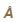and μo = 1.55 μe = 1.54. The thickness of a quater-wave plate for which μo > μe (such as calcite) is given by

Solution: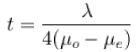Putting the given values, we get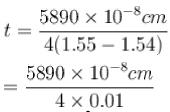= 1.47 x10-3 cm
The correct answer is: 1.47 x10-3 cm

QUESTION: 3

### Forcalcite, nO =  1.65836, ne = 1.48641 at  18°C and for λ = 5893. The thickness of the quarter wave plate is :

Solution: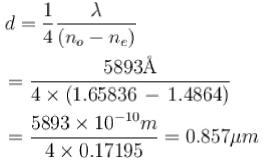The correct answer is: 0.857 μm

QUESTION: 4

Consider a plane electromagnetic wave that is a superposition of two independent orthogonal plane waves and can be written as the real part of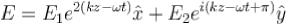If E1 = E2, the tip of the electric field vector will describe a trajectory that, is viewed along the z-axis from positive z and looking towards the origin, is a

Solution:

The correct answer is: A line 135°, to the positive x-axis

QUESTION: 5

Calculate the velocities of extraordinary rays in calcite crystal in a plane perpendicular to the optic axis. Given μO = 1.658, μ= 1.486 and c = 3 x 1010 cm/s.

Solution:

The velocity of the O-wave in the crystal is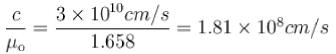= 1.81 x 108  m/s
The velocity of the E-wave in the crystal normal to be optic axis is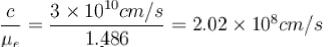= 2.02 x 10m/s
The correct answer is: = 2.02 x 10m/s

QUESTION: 6

If the plane wave is split & recombined on a screen after the two portions, which are polarized in the x & y directions, have travelled an optical path difference of 2π/k the observed average intensity will be proportional to :

Solution:

The correct answer is: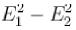QUESTION: 7

Consider a plane wave that propagates through empty space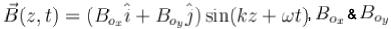are positive constants. What can you say about the polarization of this wave :

Solution:

The correct answer is: E is always parallel to the constant vector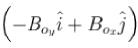QUESTION: 8

When scattered blue light is seen through a rotating nicol prism, a variation of intensity with zero minimum intensity is seen. What does this imply?

Solution:

The correct answer is: The scattered light is plane polarized

QUESTION: 9

A steady beam of light is normally incident on a piece of Polaroid. As the polarised is rotated around the beam axis, the transmitted intensity varies as A + Scos2 θ, where 6 is the angle, and A and B are constants with A > B > 0. Which of the following may be correctly concluded about the incident light?

Solution:

The correct answer is: The light is partly plane polarized and partly unpolarized

QUESTION: 10

A calcite plate 0.0031 mm thick is cut and polished such that its optic axis is parallel to the surface. A plane polarized white light from a polariser is incident normally on the plate. Estimate the phase difference if the emergent beam for wavelengths is 7068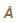, and μO = 1.6521 μe = 1.4811.

Solution:

For a negative uniaxial crystal like calcite ,μO > μe. Therefore, the path difference  between O and E-rays for calcite plate of thickness t is, Δ = ( μO- μ) t and corresponding phase difference,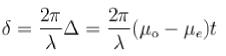Here t= 0.00031 cm
For λ = 70684,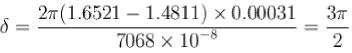As the phase difference between O and E-rays is add multiple of π/2 therefore plane polarized beam is converted into elliptically polarized light in general and circularly polarized light, if the vibration in the incident plane polarised light makes an angle of 45° with the optic axis of plate.